Get instant live expert help with Excel or Google Sheets“My Excelchat expert helped me in less than 20 minutes, saving me what would have been 5 hours of work!”

#### Post your problem and you'll get expert help in seconds

Your message must be at least 40 characters
Our professional experts are available now. Your privacy is guaranteed.

# How to Apply a Formula to Entire Column in Excel

Applying a formula is the most common task, but when we need to apply the same formula in the cells of an entire column, it becomes a tedious task. There are multiple ways to learn how to insert a formula for the entire column.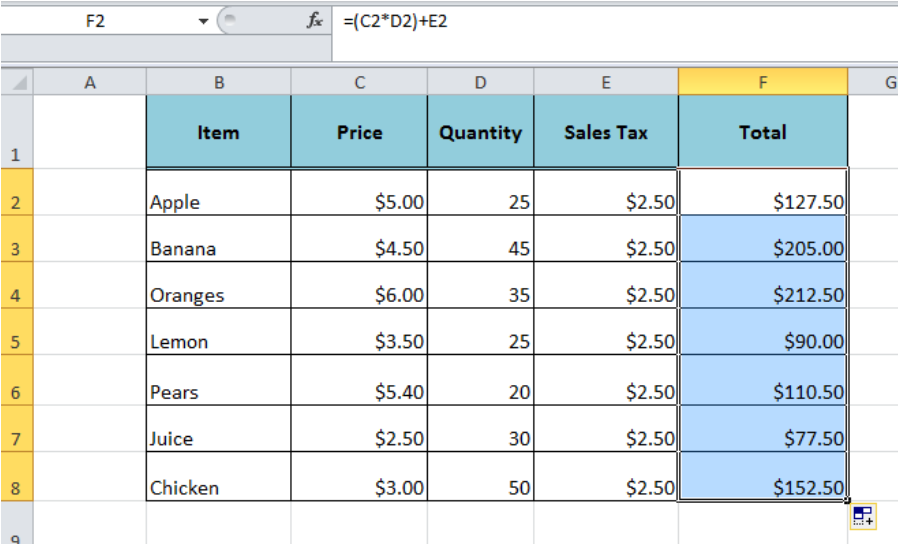Figure 1. Applying Formula to an Entire Column

Suppose we have a list of items with given price, quantity and sales tax amount and we want to calculate the total amount for each item in column F by using the formula syntax

`=(Price * Quantity) + Sales Tax`

In cell F2, we apply the formula =(C2*D2)+E2 to calculate Total Amount. There are multiple ways to learn how to apply a formula to an entire column.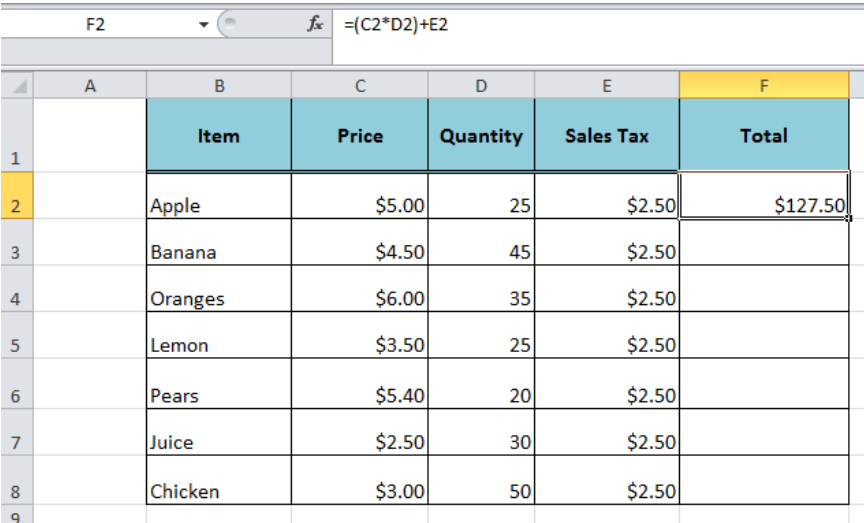Figure 2. Excel Column Functions

## By Dragging the Fill Handle

Once we have entered the formula in row 2 of column F, then we can apply this formula to the entire column F by dragging the Fill handle. Just select the cell F2, place the cursor on the bottom right corner, hold and drag the Fill handle to apply the formula to the entire column in all adjacent cells.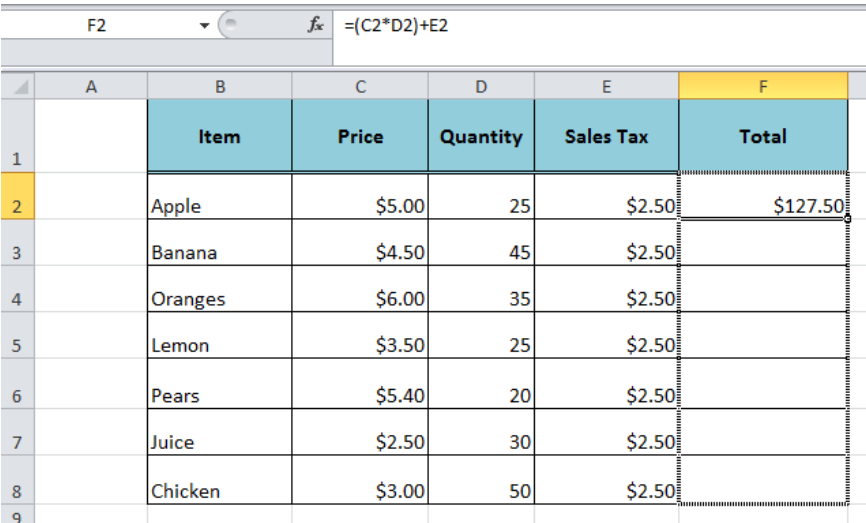Figure 3. Dragging Fill Handle

## By Double-Clicking Fill Handle

The easiest way to apply a formula to the entire column in all adjacent cells is by double-clicking the fill handle by selecting the formula cell. In this example, we need to select the cell F2 and double click on the bottom right corner. Excel applies the same formula to all the adjacent cells in the entire column F.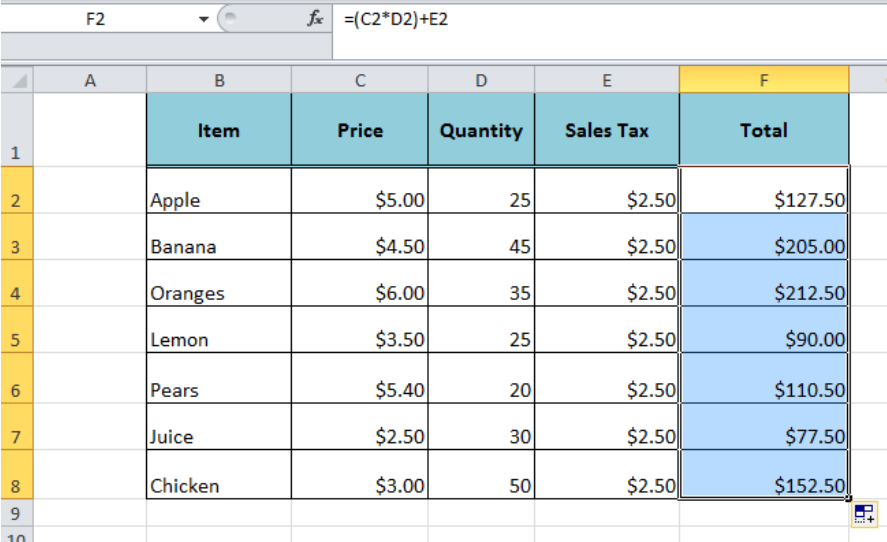Figure 4. Double Click the Fill Handle

## By Using Fill Command

Using Fill command is another good method to apply the formula to an entire column. We need to do the following to achieve for the entire column;

• After entering the formula in cell F2,
• Press Ctrl+Shift+End short keys. This will select the last used cell in the entire column.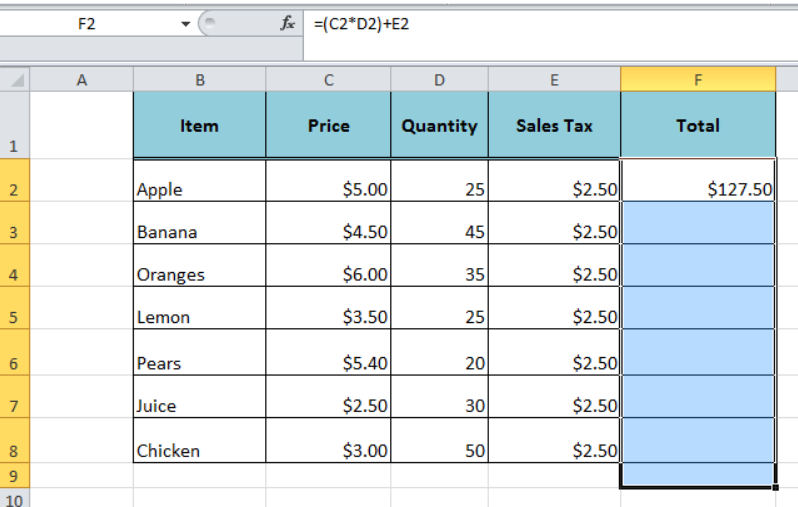Figure 5. Selecting Last Used Cell in the Entire Column

• Go to Editing Group on Home tab, click in Fill arrow and select DOWN or alternately press Ctrl+D.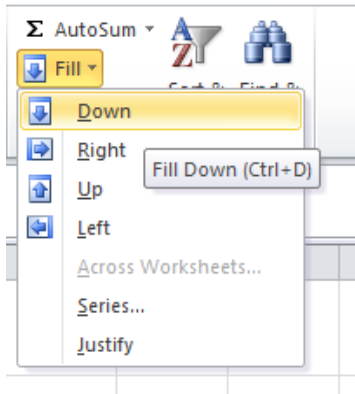Figure 6. Using Fill Command

• Fill command applies the formula to all the selected cells.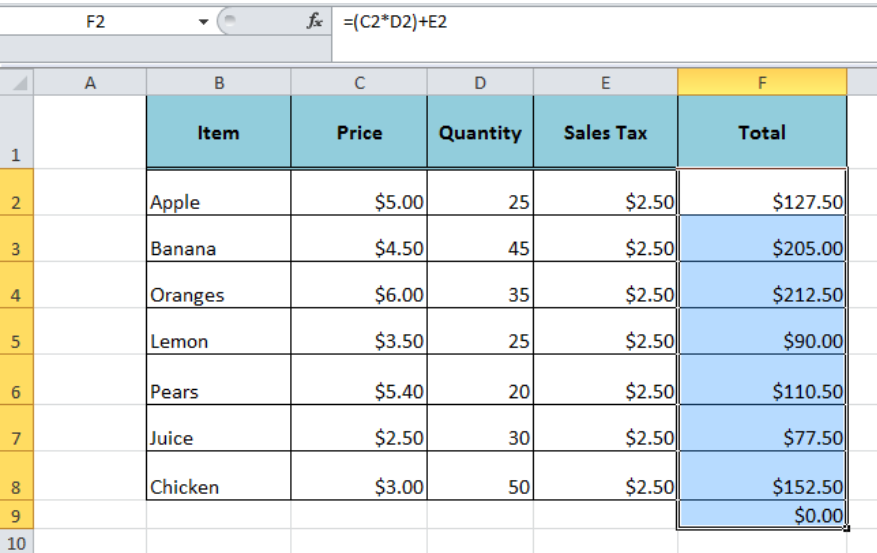Figure 7. Column Function

## Instant Connection to an Expert through our Excelchat Service

Most of the time, the problem you will need to solve will be more complex than a simple application of a formula or function. If you want to save hours of research and frustration, try our live Excelchat service! Our Excel Experts are available 24/7 to answer any Excel question you may have. We guarantee a connection within 30 seconds and a customized solution within 20 minutes.